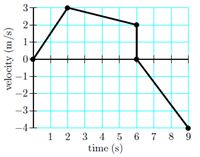# Problem: Consider the plot describing motion along a straight line with an initial position of 10 m. What is the position at 9 seconds? 1. 30.0 2. 24.0 3. 49.0 4. 42.0 5. 29.0 6. 31.5 7. 12.0 8. 17.0 9. 41.0 10. 21.5

###### FREE Expert Solution
95% (358 ratings)
###### Problem Details

Consider the plot describing motion along a straight line with an initial position of 10 m. What is the position at 9 seconds?

1. 30.0

2. 24.0

3. 49.0

4. 42.0

5. 29.0

6. 31.5

7. 12.0

8. 17.0

9. 41.0

10. 21.5Frequently Asked Questions

What scientific concept do you need to know in order to solve this problem?

Our tutors have indicated that to solve this problem you will need to apply the Calculating Displacement from Velocity-Time Graphs concept. You can view video lessons to learn Calculating Displacement from Velocity-Time Graphs. Or if you need more Calculating Displacement from Velocity-Time Graphs practice, you can also practice Calculating Displacement from Velocity-Time Graphs practice problems.

How long does this problem take to solve?

Our expert Physics tutor, Jeffery took 2 minutes and 45 seconds to solve this problem. You can follow their steps in the video explanation above.

What professor is this problem relevant for?

Based on our data, we think this problem is relevant for Professor Mezonlin's class at FAMU.